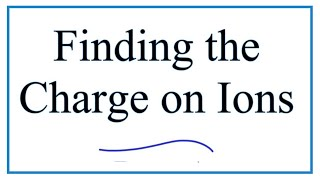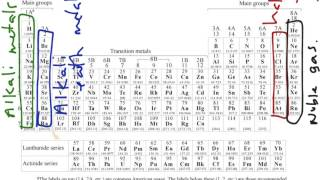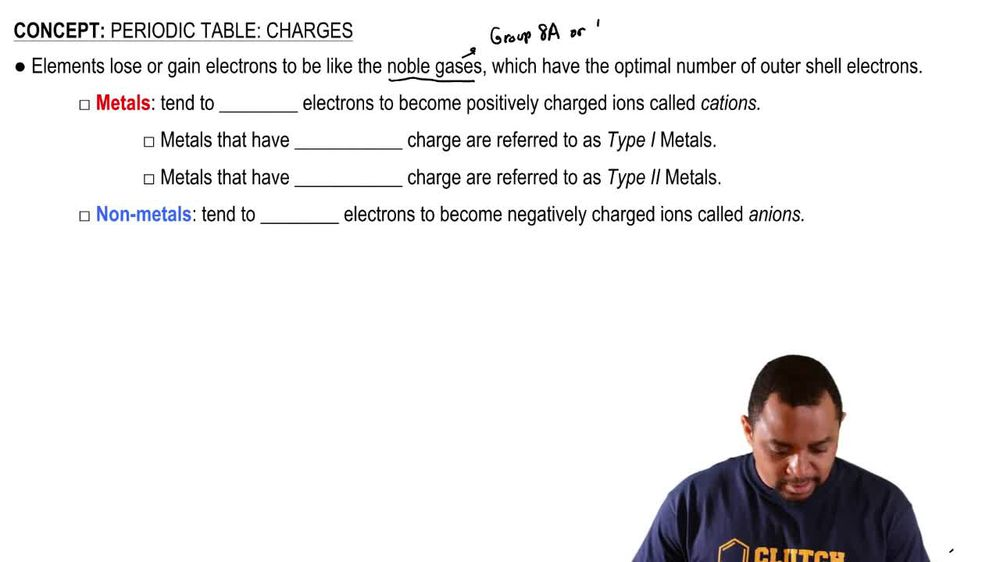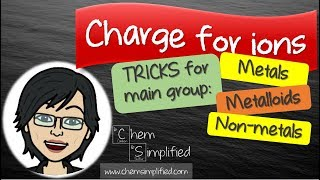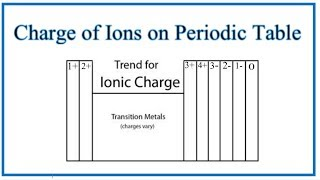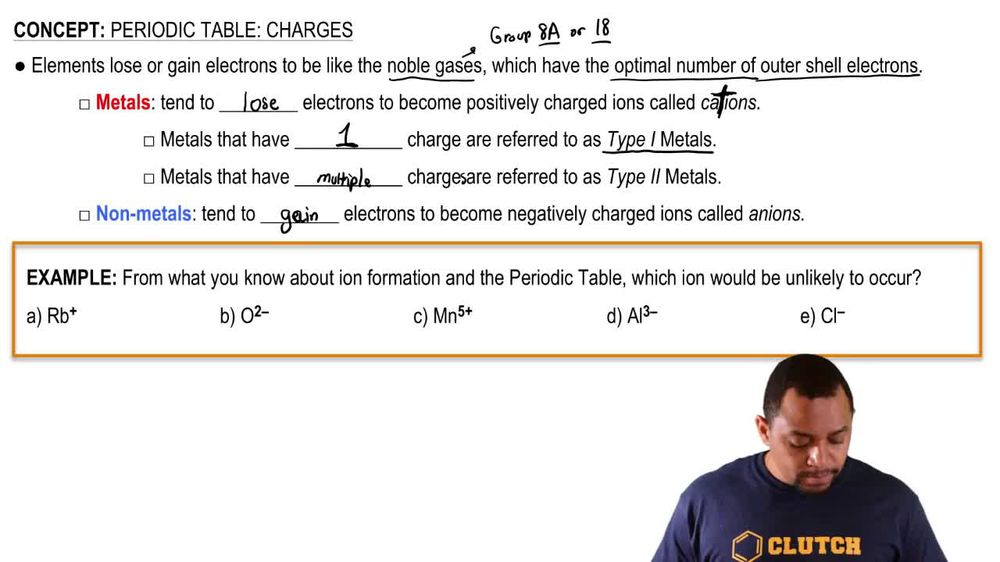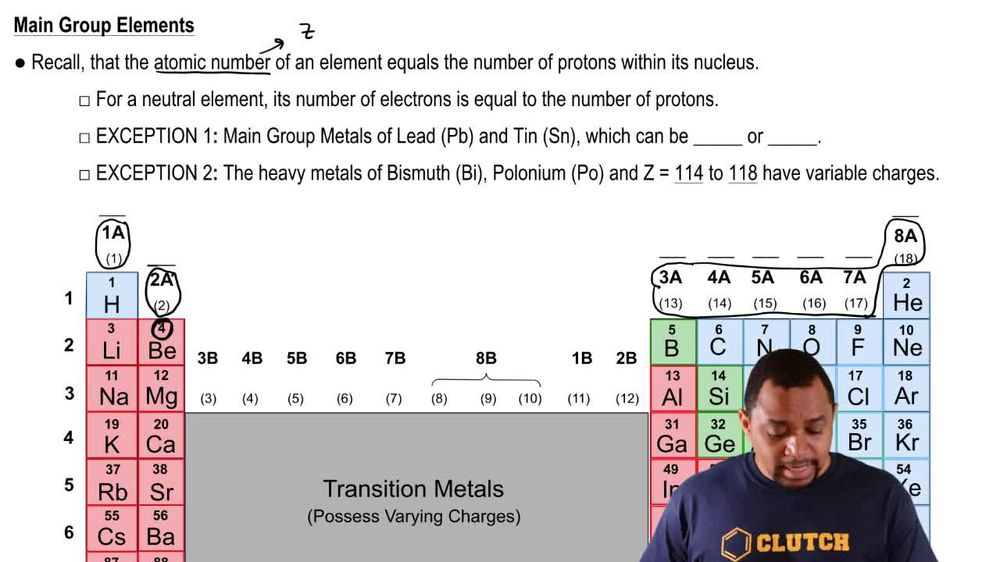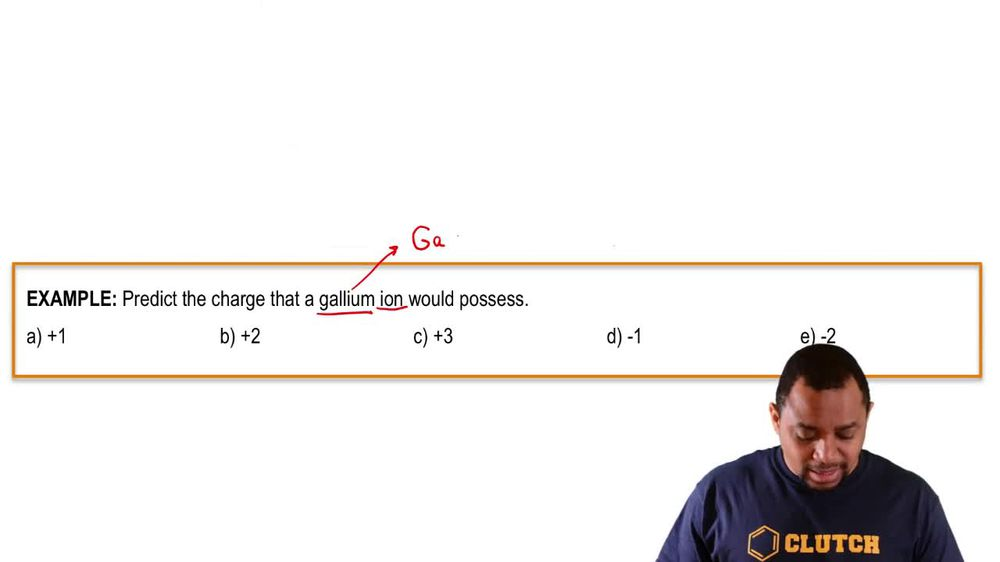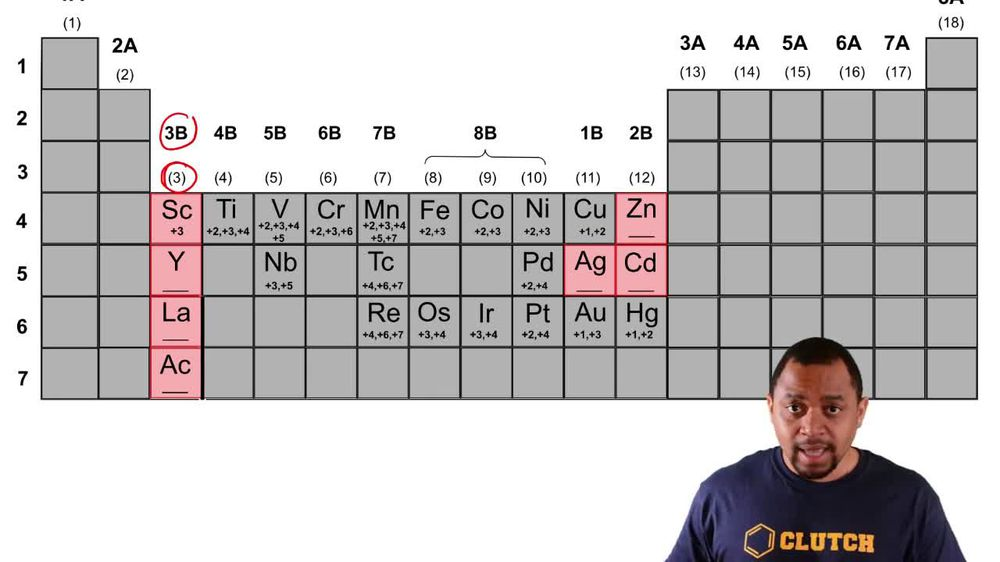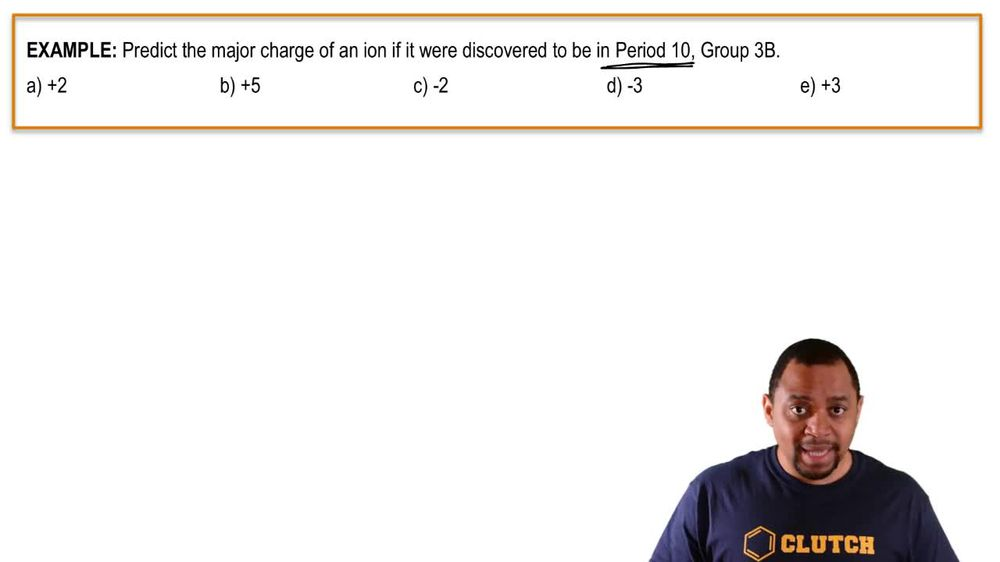Start typing, then use the up and down arrows to select an option from the list.
1. 2. Atoms & Elements2. Periodic Table: Charges
Problem

# Using the periodic table, predict the charge of the most stable ion of the following elements: (d) I

Relevant Solution1m
Play a video:
Hey everyone in this example, we need to locate the below elements on the periodic table and identify the charge of their ions. So looking at our first given atom sodium, we would recall that sodium is located in Group one A. Of our periodic table. And we should recall that the group number corresponds to our ion charge. So we should recall that any atom or element in Group one A. Is going to form a plus one cat ion. And so therefore we would form the N. A. Plus cat eye on. So this is going to be our answer for part A. And now we're going to move forward to part B. So in part B. We're given the atom bombing. When we find roaming on the periodic table. We see that it's in group seven A. And we would recall that any atom and group seven A. Should have A minus one an ion charge. And so therefore we would form the B. R minus an ion. And so this is our second answer for part B. Moving on to part C. We have the atom nitrogen. When we find nitrogen on the periodic table, we see that nitrogen is located in group five A. We would respond that. Or we would recall that any atom and Group five A. Is going to form the minus three an ion charge. And so therefore we would say we would form the N three minus an eye on. So this is our answer for part C. And moving on to part D. Of this example, we have the atom calcium. We recall adam we recall the calcium atoms location on our periodic table in Group two A. Which we should recall. Any atom in Group two A. Will form a plus two cat ion charge because it's positive and so therefore we would form the C. A. Two plus cat eye on. So this is our answer for part D. Everything boxed in blue represents the ions that we would form from the given atoms as our final answers. So if you have any questions, please leave them down below. Otherwise, I'll see everyone in the next practice video.